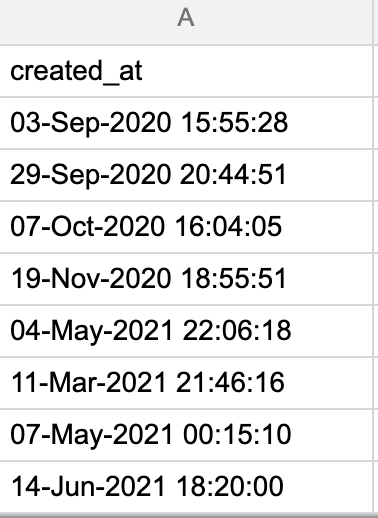I recently posted a question here about how to create a time series that includes all month periods and got a helpful answer, but now I'm stuck on a variation of that that I'm hoping to make a graph showing growth of the same data over time. Again the data looks like this:What I'd like is a graph that shows each month over the last couple of years where for each month it shows the total number of rows where the date is prior to the end of that month. So, in this example if would show 2 for September 2020, 3 for October 2020, 4 for November 2020, and then 4 for each month until March 2021. The previous answer used the LOOKUP function, but I need something like "for each value you're looking up, find the count of rows where the value is less than or equal to that", and it seems like maybe this is not possible with LOOKUP. Thanks in advance!